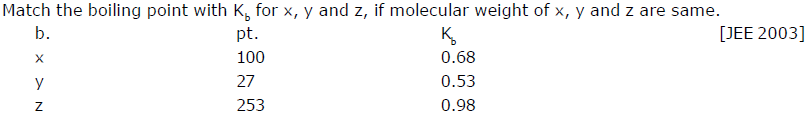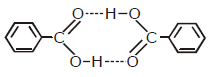Courses

# Previous Year Questions - Solutions (IIT-JEE Advanced) JEE Notes | EduRev

## JEE : Previous Year Questions - Solutions (IIT-JEE Advanced) JEE Notes | EduRev

The document Previous Year Questions - Solutions (IIT-JEE Advanced) JEE Notes | EduRev is a part of the JEE Course Chemistry for JEE Advanced.
All you need of JEE at this link: JEE

Q 1.  To 500 cm3 of water, 3 × 10-3 kg of acetic is added. If 23% of acetic acid is dissociated, what will be the depression in freezing point? Kf and density of water are 1.86 K kg-1 mol-1 and 0.997 g cm-3 respectively.

[JEE 2000]

Ans.  0.229

Q 2.  The vapour pressure of two miscible liquids (A) and (B) are 300 and 500 mm of Hg respectively. In a flask 10 mole of (A) is mixed with 12 mole of (B). However, as soon as (B) is added, (A) starts polymerising into a completely insoluble solid. The polymerisation follows first-order kinetics. after 100 minute, 0.525 mole of a solute is dissolved which arrests the polymerisation completely. The final vapour pressure of the solution is 400 mm of Hg. Estimate the rate constant of the polymerisation reaction. Assume negligible volume change on mixing and polymerisation and ideal behaviour for the final solution. [JEE 2001]

Ans. 1.0×10-4

Q 3.Ans. Kb(x) = 0.68, Kb(y) =0.53, Kb(z) = 0.98

Q 4. 1.22 g of benzoic acid is dissolved in

(i) 100 g acetone (Kb for acetone = 1.7) and

(ii) 100 g benzene (Kb for benzene = 2.6). The elevation in boiling points Tb is 0.17ºC and 0.13ºC respectively. [JEE 2004]

(a) What are the molecular weights of benzoic acid in both the solutions?

(b) What do you deduce out of it in terms of structure of benzoic acid?

Ans. (a) 122,

(b) It means that benzoic acid remains as it is in acetone while it dimerises in benzene asQ 5. 72.5 g of phenol is dissolved in 1 kg of a solvent (kf = 14) which leads to dimerization of phenol and freezing point is lowered by 7 kelvin. What percent of total phenol is present in dimeric form?     [JEE 2006]

Ans. 35% phenol is present in dimeric form

Offer running on EduRev: Apply code STAYHOME200 to get INR 200 off on our premium plan EduRev Infinity!

53 docs|118 tests

,

,

,

,

,

,

,

,

,

,

,

,

,

,

,

,

,

,

,

,

,

;